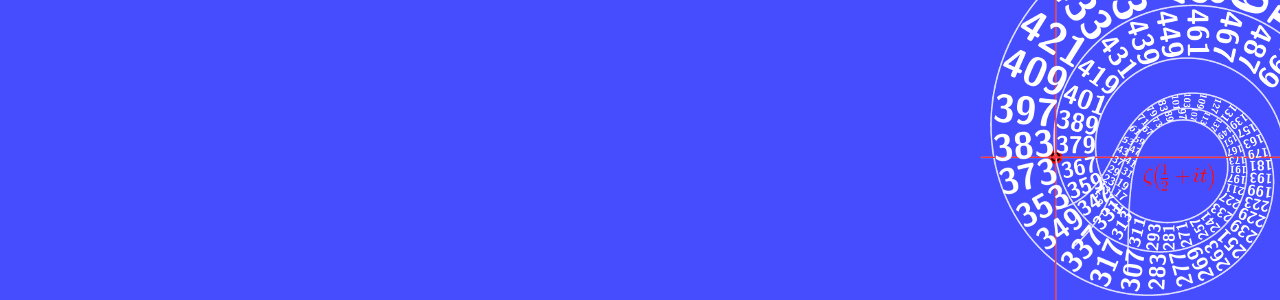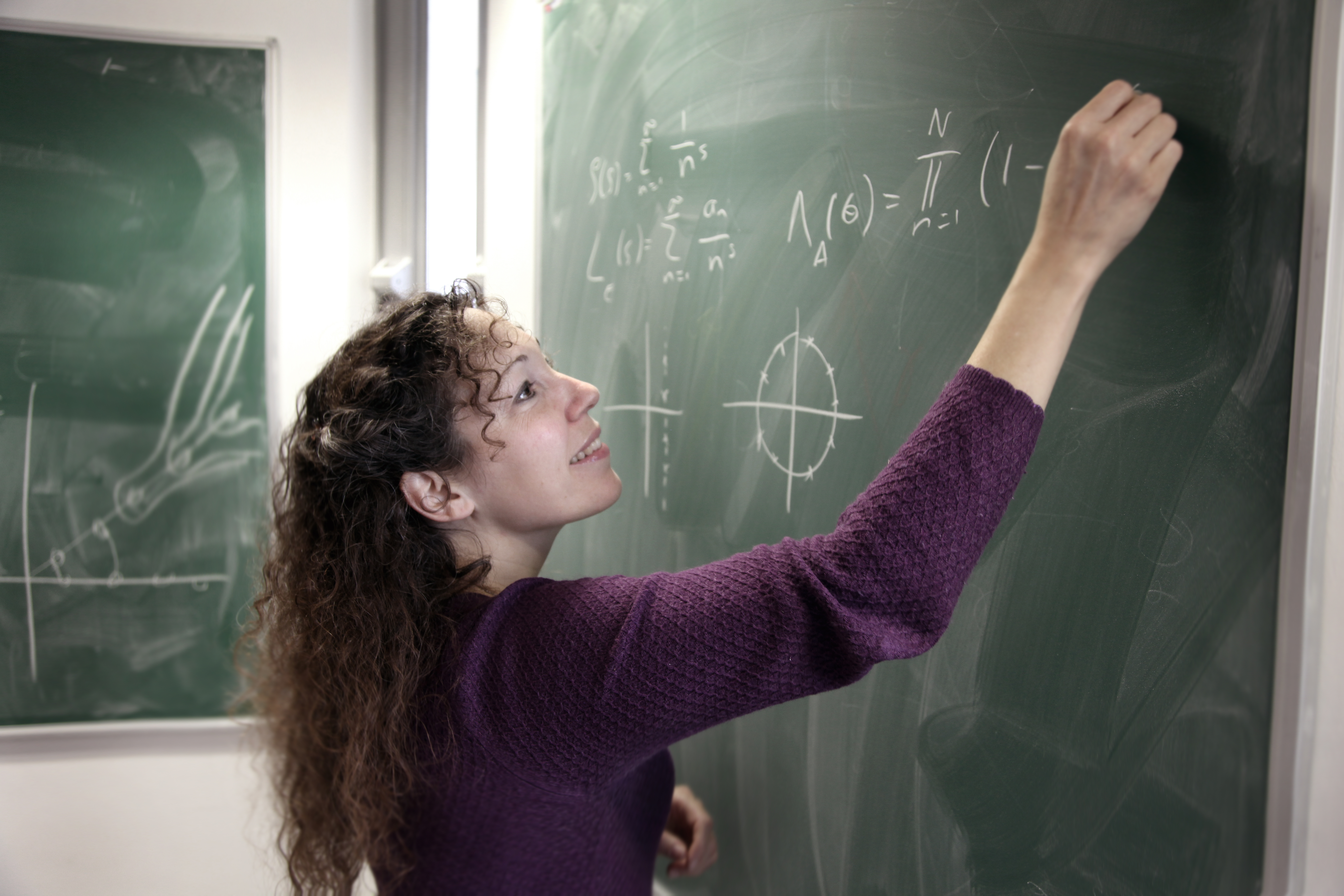Zeta functions in number theory
and mathematical physics
LMS Regional Meeting
Nottingham 2019
LMS Meeting Nottingham 2019
LMS Meeting Nottingham 2019

Wednesday, September 11, 2019

Zeta functions in number theory and mathematical physics

## SpeakersJohn Coates
(Cambridge)Nina Snaith
(Bristol)Mark Pollicott
(Warwick)

## Programme

The lectures took place in the room B13 in the Physics building.
See here for directions.
For the programme of the following workshop see here.

• 1:30 : Opening of the Meeting & Society Business
• 2:00 : Nina Snaith:
"Random matrix theory, the Riemann zeta function and elliptic curves"
• 3:00 : Coffee break
• 3:30 : John Coates:
"$$L$$-values and the exact Birch-Swinnerton-Dyer formula"
• 4:40 : Mark Pollicott:
"Dynamical zeta functions and their applications"
• 5:40 : Wine reception in the atrium of the maths building
• 7:00 : Dinner at the Victoria in Beeston

## Abstracts

Here the abstracts fro the three talks.

Nina Snaith:
"Random matrix theory, the Riemann zeta function and elliptic curves"

The study of eigenvalue statistics of random matrices emerged in nuclear physics in the 1950's and 60's. However through the similarity of these statistics with those of the zeros of number theoretical functions, applications of random matrix theory to number theory vary from moments and distributions of $$L$$-functions to investigating ranks of elliptic curves and surprisingly detailed studies of the Riemann zeta function, its zeros and its derivative. This talk will highlight some of these results.

John Coates:
"$$L$$-values and the exact Birch-Swinnerton-Dyer formula."

Let $$K = \mathbb{Q}(\sqrt{-q})$$, where $$q$$ is a prime $$\equiv 7 \mod 8$$, and let $$h$$ be the class number of $$K$$. One of the important achievements of the great 19th century German school of number theory was Dirichlet's proof, using the value at $$s=0$$ of his $$L$$-function $$L(\chi, s)$$ attached to $$K$$, that $$h = R - N$$, where $$R$$ is the number of residues and $$N$$ is the number of non-residues modulo $$q$$ in the set $$\{1, 2, \dots, (q-1)/2 \}$$. No proof of even the assertion that always $$R > N$$ which does not involve $$L$$-values has ever been found. As we shall explain in more detail in the lecture, a special case of the celebrated conjecture of Birch and Swinnerton-Dyer predicts a precise analogue of Dirichlet's formula for elliptic curves $$E$$, which are defined over the Hilbert class field $$H$$ of $$K$$, and admit complex multiplication by the ring of integers of $$K$$. Indeed, thanks to a theorem of Deuring, the complex $$L$$-series $$L(E/H,s)$$ attached to such an $$E$$ has an analytic continuation over the whole complex plane, and, in particular, the conjecture of Birch and Swinnerton-Dyer asserts that $$L(E/H, 1) \neq 0$$ if and only if the group $$E(H)$$ of $$H$$-rational points on $$E$$ is finite. In my lecture, I hope to explain some joint work with Y. Li which proves that $$L(E/H, 1) \neq 0$$ for a large explicit infinite class of such $$E$$. Moreover, for this same large class of $$E$$ with $$L(E/H, 1) \neq 0$$, I will discuss ongoing work with Y. Kezuka, Y. Li, and Y. Tian whose aim is to prove that the Tate-Shafarevich group Ш$$(E/H)$$ is indeed finite and has order exactly as predicted by the conjecture of Birch and Swinnerton-Dyer. I shall also discuss some numerical illustrations of these results. The theoretical arguments will all be based on Iwasawa theory.

Mark Pollicott:
"Dynamical zeta functions and their applications"

We will describe how the famous Riemann zeta function inspired zeta functions in geometry and dynamical systems and describe results on their domains. We will also discuss a possibly slightly surprising application to the Zaremba conjecture in number theory.

## Organisation

The local organisers:

Explanations of how to reach the University of Nottingham, information about accommodation and other helpful things can be found here.